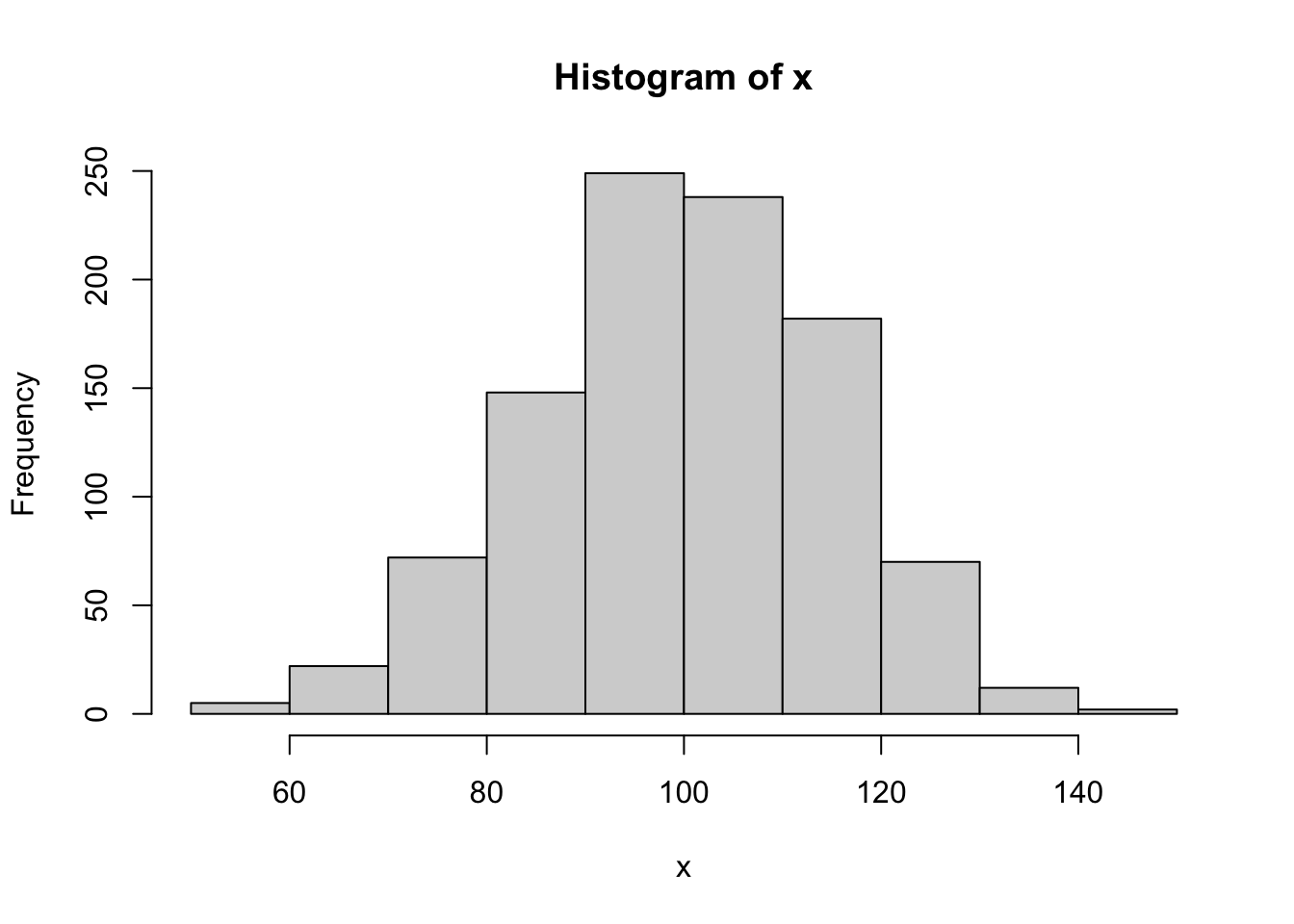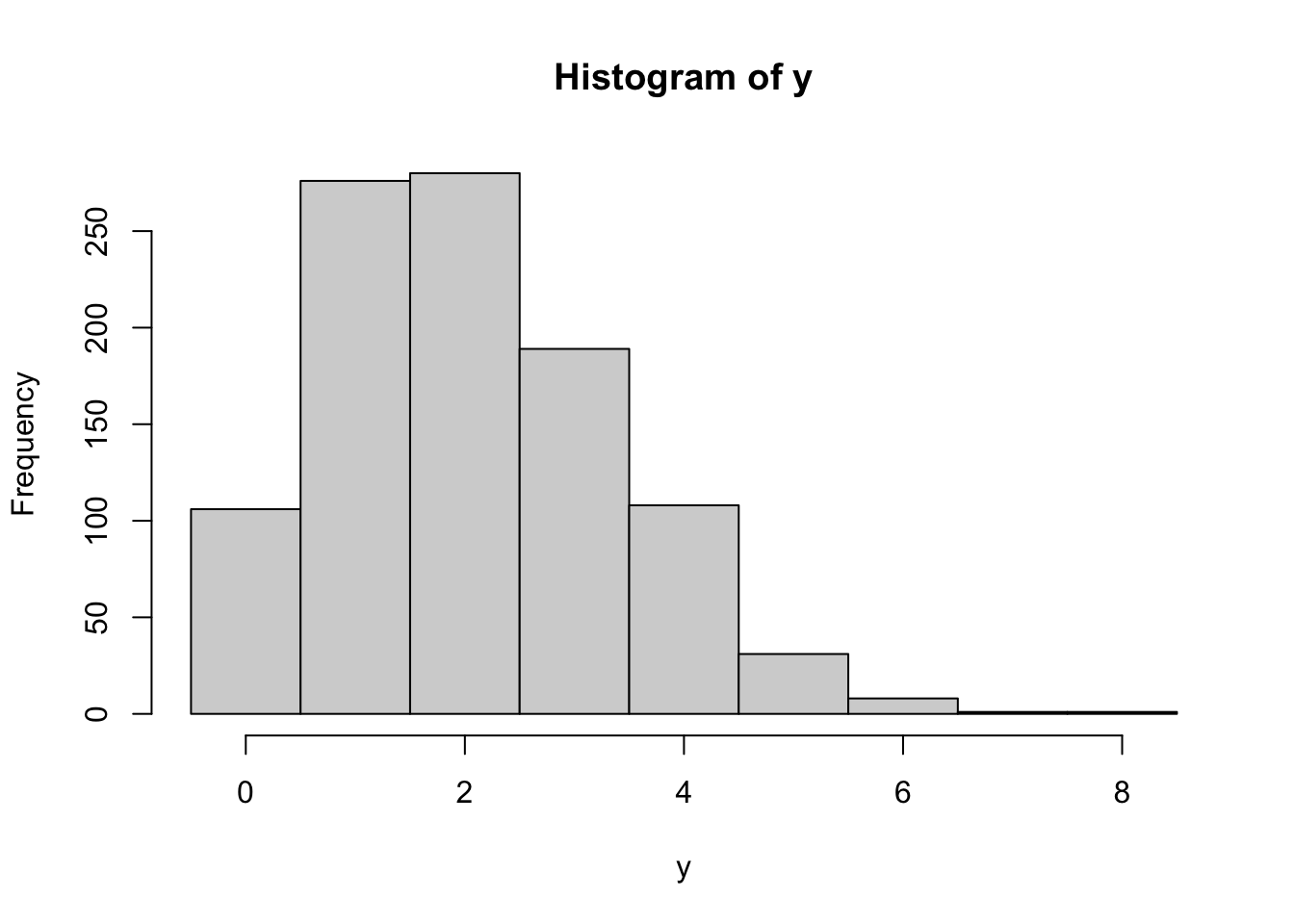The rand_bytes function binds to RAND_bytes in OpenSSL to generate cryptographically strong pseudo-random bytes. See the OpenSSL documentation for what this means.

rnd <- rand_bytes(10)
print(rnd)
  19 26 12 10 48 26 d2 6d 5f 78

Bytes are 8 bit and hence can have 2^8 = 256 possible values.

as.numeric(rnd)
   25  38  18  16  72  38 210 109  95 120

Each random byte can be decomposed into 8 random bits (booleans)

x <- rand_bytes(1)
as.logical(rawToBits(x))
  TRUE  TRUE FALSE FALSE  TRUE  TRUE  TRUE  TRUE

## Secure Random Numbers

rand_num is a simple (2 lines) wrapper to rand_bytes to generate random numbers (doubles) between 0 and 1.

rand_num(10)
  0.5267039 0.6312105 0.3572912 0.8131160 0.8826681 0.3573297 0.9870108
 0.6157770 0.7853889 0.4496631

To map random draws from [0,1] into a probability density, we can use a Cumulative Distribution Function. For example we can combine qnorm and rand_num to simulate rnorm:

# Secure rnorm
x <- qnorm(rand_num(1000), mean = 100, sd = 15)
hist(x)Same for discrete distributions:

# Secure rbinom
y <- qbinom(rand_num(1000), size = 20, prob = 0.1)
hist(y, breaks = -.5:(max(y)+1))